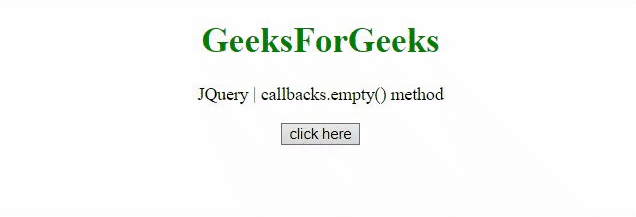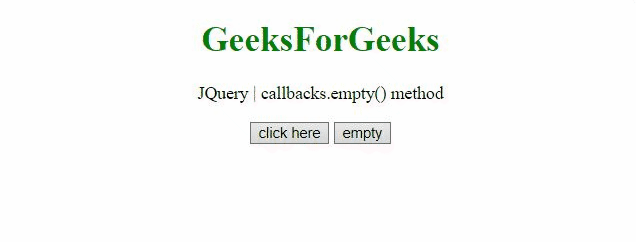# jQuery callbacks.empty() Method

The callbacks.empty() method in jQuery is used to remove all the callbacks from a list. It returns the Callbacks object onto which it is attached.

Syntax:

`callbacks.empty()`

Parameters: It does not accept any parameter.

Return Value: This method returns the Callbacks object onto which it is attached.

Below examples illustrate the callbacks.empty() method in jQuery:

Example 1: In this example, the fun1 function is first added to the callbacks list and all the callbacks present are executed in the list with a given argument. The callbacks.empty() method is executed to empty the list. The second function fun2 is added and the callbacks list is executed again. This demonstrates the emptying of the list after adding the first function.

## html

 ` ` `<``html``> ` ` `  `<``head``> ` `    ``<``title``> ` `        ``JQuery callbacks.empty() method ` `    `` ` ` `  `    ``<``script` `src``= ` `"https://code.jquery.com/jquery-3.5.0.js"``> ` `    `` ` ` ` ` `  `<``body` `style``=``"text-align:center;"``> ` ` `  `    ``<``h1` `style``=``"color:green;"``> ` `        ``GeeksForGeeks ` `    `` ` ` `  `    ``<``p``> ` `        ``JQuery | callbacks.empty() method ` `    `` ` ` `  `    ``<``button` `onclick``=``"Geeks();"``> ` `        ``click here ` `    `` ` ` `  `    ``<``p` `id``=``"output"``> ` ` `  `    ``<``script``> ` `        ``var output = document.getElementById("output"); ` `        ``var res = ""; ` ` `  `        ``// Initialize a callback list ` `        ``var callbacks = jQuery.Callbacks(); ` ` `  `        ``function Geeks() { ` `             `  `            ``// First function to be added to the list ` `            ``var fun1 = function (val) { ` `                ``res = res + "This is function 1 and" + ` `                    ``" value passed is " + val + "<``br``>"; ` `            ``}; ` ` `  `            ``// Second function to be added to the list ` `            ``var fun2 = function (val) { ` `                ``res = res + "This is function 2 and" + ` `                    ``" value passed is " + val + "<``br``>"; ` `            ``}; ` ` `  `            ``// Adding the first function ` `            ``callbacks.add(fun1); ` ` `  `            ``// Calling the first function ` `            ``callbacks.fire("GFG_1"); ` ` `  `            ``// Clearing the callback list ` `            ``callbacks.empty(); ` ` `  `            ``// Adding the second function ` `            ``callbacks.add(fun2); ` ` `  `            ``// Calling the first function ` `            ``callbacks.fire("GFG_2"); ` ` `  `            ``output.innerHTML = res; ` `        ``}  ` `    `` ` ` ` ` `  ` `

Output:Example 2: This example provides a button to empty the callback list and then add the given function to see the result of emptying the callbacks list.

## html

 ` ` `<``html``> ` ` `  `<``head``> ` `    ``<``title``> ` `        ``JQuery | callbacks.empty() method ` `    `` ` ` `  `    ``<``script` `src``= ` `"https://code.jquery.com/jquery-3.5.1.min.js"``> ` `    `` ` ` ` ` `  `<``body` `style``=``"text-align:center;"``> ` ` `  `    ``<``h1` `style``=``"color:green;"``> ` `        ``GeeksForGeeks ` `    `` ` ` `  `    ``<``p``> ` `        ``JQuery | callbacks.empty() method ` `    `` ` ` `  `    ``<``button` `onclick``=``"Geeks();"``> ` `        ``click here ` `    `` ` ` `  `    ``<``button` `onclick``=``"empty();"``> ` `        ``empty ` `    `` ` `     `  `    ``<``p` `id``=``"output"``> ` ` `  `    ``<``script``> ` `        ``var output = document.getElementById("output"); ` `        ``var res = ""; ` ` `  `        ``// Initialize a callback list ` `        ``var callbacks = jQuery.Callbacks(); ` ` `  `        ``function empty() { ` ` `  `            ``// Clear the callback list ` `            ``callbacks.empty(); ` `        ``} ` ` `  `        ``// Function to add and fire callbacks  ` `        ``function Geeks() { ` ` `  `            ``// Function to be added to the list ` `            ``var fun1 = function (val) { ` `                ``res = res + "This is function 1 and" + ` `                    ``" value passed is " + val + "<``br``>"; ` `            ``}; ` ` `  `            ``// Adding the given function ` `            ``callbacks.add(fun1); ` ` `  `            ``// Calling the function with value ` `            ``callbacks.fire("GFG_1"); ` ` `  `            ``output.innerHTML = res; ` `        ``}  ` `    `` ` ` ` ` `  ` `

Output:My Personal Notes arrow_drop_upCheck out this Author's contributed articles.

If you like GeeksforGeeks and would like to contribute, you can also write an article using contribute.geeksforgeeks.org or mail your article to contribute@geeksforgeeks.org. See your article appearing on the GeeksforGeeks main page and help other Geeks.

Please Improve this article if you find anything incorrect by clicking on the "Improve Article" button below.

Article Tags :

Be the First to upvote.

Please write to us at contribute@geeksforgeeks.org to report any issue with the above content.